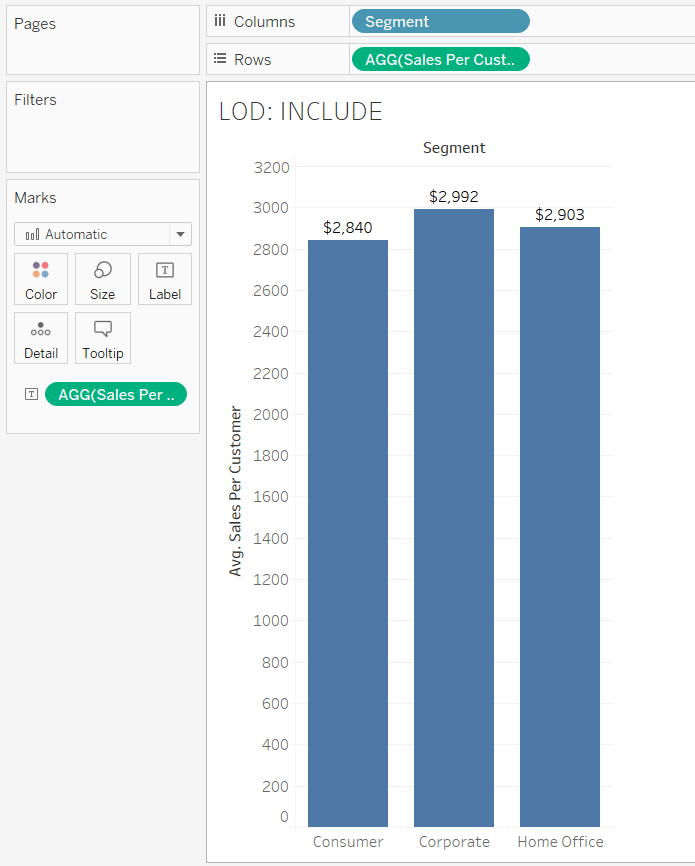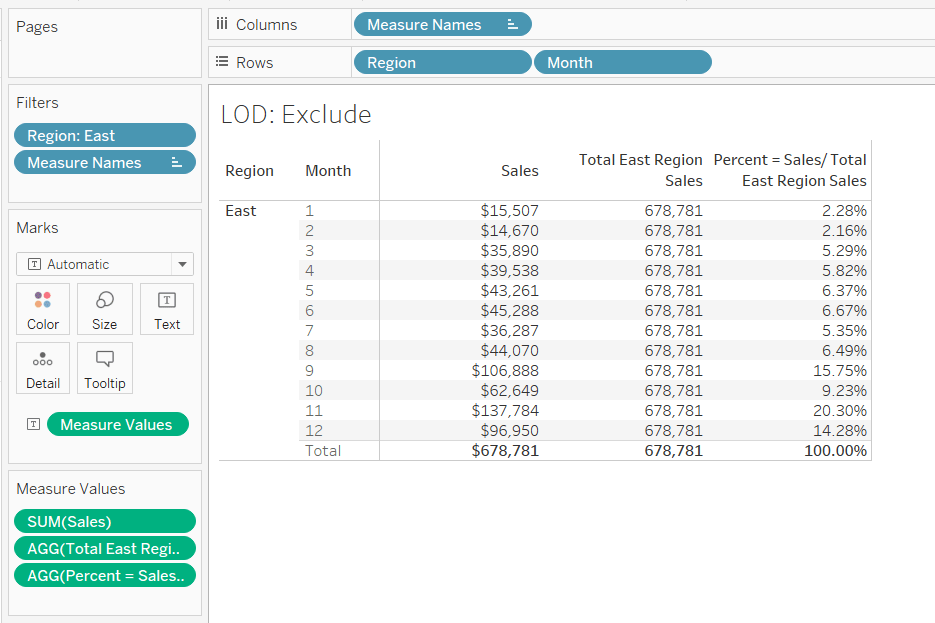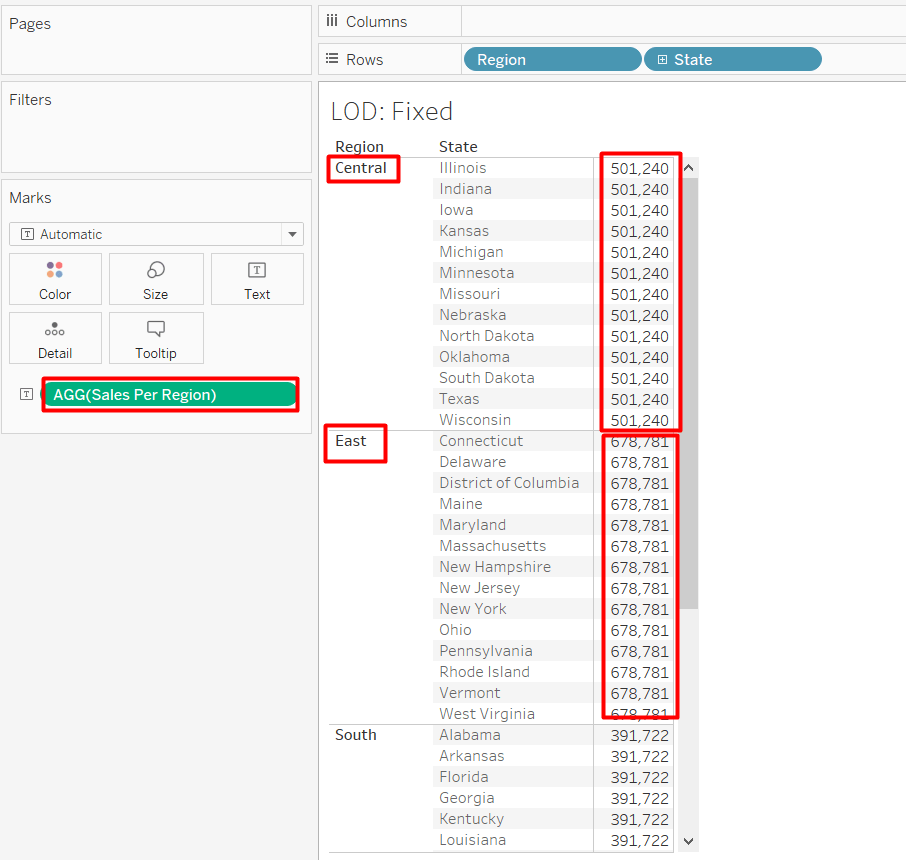• Home  /

# Super Easy Guide to Tableau LOD Calculations

Level of Details (LOD’s) Expressions in Tableau

Using LOD expressions, a user can compute different levels of aggregations that are not at the level of detail of the visualization. If you want to improve your Tableau skills check out: Best Tableau Online Courses.

LOD’s Expressions:

In Tableau, we have 3 types of Level of Details expressions. These are “INCLUDE”, “EXCLUDE” and “FIXED”.

The following is a detailed explanation of each of the LOD expression with examples:

## I. INCLUDE

As the name suggests, “INCLUDE” level of detail expression compute aggregations considering dimensions which are specified in the calculation and also take into consideration those dimensions which are present in the view. Check out this tutorial for a Deep Dive into INCLUDE LOD calculation.

“INCLUDE” level of detail expression is used when a user wants to calculate values at the lower level of granularity and then aggregate and show the values at the higher level of granularity.

Example:
Let’s consider the requirement is to compare the average customer sales across different product segments.

In this case, although in the view we are going to show only “Segment” in calculations we need to include “Customer Name” as well so that average customer sales can be calculated.

`Formula/Expression:  AVG({INCLUDE [Customer Name] : SUM([Sales])})`

Explanation: In the above formula, we are including the lower level of dimension i.e. Customer Name to get the aggregate (sum) values of Sales at that level and then we are showing the average at higher level i.e. Segment

Tableau View:## II. EXCLUDE

“EXCLUDE” level of detail expression is used to omit specified dimensions from the aggregations. Using “EXCLUDE”, the user can omit the lower level granularity dimension which is present in the view and can directly calculate the value at higher granularity level. If you need more detail check out,  EXCLUDE Deep Dive.

“EXCLUDE” level of detail expression is majorly used to calculate ‘difference from overall average’ or ‘percent of total’.

Example:
Let’s consider the requirement is to compare total sales with the monthly sales of the East region.

To get the monthly sales we need to have “Region”, “Month” and “Sum of Sales” in the view. But, now to get the total sales of Region, we need to exclude “Month” in the calculation so that we can have total sales value for the region.

`Formula/Expression:  SUM({EXCLUDE [Month] : SUM([Sales])})`

Explanation: In the above formula, we are excluding the lower level of dimension i.e. Month to get the aggregate (sum) values of Sales at a higher level i.e. Region.

Tableau View:## III. FIXED

“FIXED” level of detail expression aggregates the value only at the dimensions which are specified by the user in the calculation. “FIXED” expression does not take into consideration those dimensions in the view. If you need more detail, check out a LOD FIXED Deep Dive.

Example:
Let’s consider the following expression which calculates the sales across regions.

`Formula/Expression:  SUM({FIXED [Region] : SUM([Sales])})`

Explanation: The above calculation will show the sales value across the region. The objective of using FIXED is that even if a user puts another field say “State” in the view, the above calculation still calculates the value at region level only. It will disregard the other dimensions which are in the view i.e. State

Tableau View: